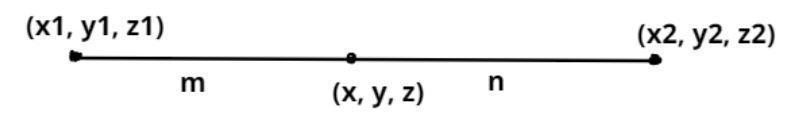Courses
Courses for Kids
Free study material
Free LIVE classes
MoreLIVE
Join Vedantu’s FREE Mastercalss

# The point which divides the line joining the points $\left( {1,3,4} \right)$ and $\left( {4,3,1} \right)$ internally in the ratio$2:1$ is$a.\left( {2, - 3,3} \right) \\ b.\left( {2,3,3} \right) \\ c.\left( {\dfrac{5}{2},3,\dfrac{5}{2}} \right) \\ d.\left( { - 3,3,2} \right) \\ e.\left( {3,3,2} \right) \\$Verified
363k+ views
Hint: Apply section formula if a line divide the points $\left( {{x_1},{y_1},{z_1}} \right)$ and $\left( {{x_2},{y_2},{z_2}} \right)$ in the ratio $\left( {m:n} \right)$ internally then
$x = \dfrac{{\left( {m.{x_2} + n.{x_1}} \right)}}{{m + n}},y = \dfrac{{\left( {m.{y_2} + n.{y_1}} \right)}}{{m + n}},z = \dfrac{{\left( {m.{y_2} + n.{y_1}} \right)}}{{m + n}}$Given points are $\left( {1,3,4} \right)$ and $\left( {4,3,1} \right)$.
Let $\left( {1,3,4} \right) \equiv \left( {{x_1},{y_1},{z_1}} \right)$
And $\left( {4,3,1} \right) \equiv \left( {{x_2},{y_2},{z_2}} \right)$
Now, let the point $\left( {x,y,z} \right)$ divide the line internally in the ratio $2:1$
So, by section formula if a line divide the points $\left( {{x_1},{y_1},{z_1}} \right)$ and $\left( {{x_2},{y_2},{z_2}} \right)$ in the ratio $\left( {m:n} \right)$ internally then
$x = \dfrac{{\left( {m.{x_2} + n.{x_1}} \right)}}{{m + n}},y = \dfrac{{\left( {m.{y_2} + n.{y_1}} \right)}}{{m + n}},z = \dfrac{{\left( {m.{y_2} + n.{y_1}} \right)}}{{m + n}}$
Here $m = 2{\text{ & }}n = 1$
$x = \dfrac{{\left( {2.\left( 4 \right) + 1.\left( 1 \right)} \right)}}{{2 + 1}},y = \dfrac{{\left( {2.\left( 3 \right) + 1.\left( 3 \right)} \right)}}{{2 + 1}},z = \dfrac{{\left( {2.\left( 1 \right) + 1.\left( 4 \right)} \right)}}{{2 + 1}}$
$\Rightarrow \left( {x,y,z} \right) = \left( {\dfrac{9}{3},\dfrac{9}{3},\dfrac{6}{3}} \right) = \left( {3,3,2} \right)$

Hence option (e) is correct.

Note: In such types of questions the key concept we have to remember is that always recall the section formula which is stated above then substitute the given points in this formula and simplify, we will get the required points which divide the line internally in the ratio $2:1$.
Last updated date: 23rd Sep 2023
Total views: 363k
Views today: 5.63k